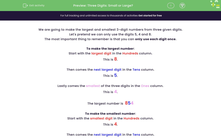# Make the smallest and largest 3 digit numbers

In this worksheet, students are given three digits to arrange into the largest and smallest possible numbers.Key stage:  KS 2

Curriculum topic:   Number: Number and Place Value

Curriculum subtopic:   Order/Compare Numbers to 1000

Difficulty level:#### Worksheet Overview

In this activity, we are going to learn to make the largest and smallest 3-digit numbers from three given digits.

Using only the digits 5, 4, and 8, we need to create the largest and smallest 3-digit numbers possible..

We can only use each digit once.

To make the largest number:

This is 8.

Then comes the next largest digit in the Tens place.

This is 5.

Lastly comes the smallest of the three digits in the Ones place.

This is 4.

The largest possible number is  854

To make the smallest number:

This is 4.

Then comes the next largest digit in the Tens place.

This is 5.

Lastly comes the largest of the three digits in the Ones place.

This is 8.

The smallest number is  458.

Now that you've been shown the method to use, let;s try an example question together.

Example

Use each of the digits:

686

to form the smallest and largest 3-digit numbers that you can make.

6 is the smallest digit.

6 is the "middle" digit even though it is the same as the smallest here.

8 is the largest digit.

The smallest number is 668.

The largest number is 866.

### What is EdPlace?

We're your National Curriculum aligned online education content provider helping each child succeed in English, maths and science from year 1 to GCSE. With an EdPlace account you’ll be able to track and measure progress, helping each child achieve their best. We build confidence and attainment by personalising each child’s learning at a level that suits them.

Get started